# NR PDSCH Throughput Using Channel State Information Feedback

This reference simulation measures the physical downlink shared channel (PDSCH) throughput of a 5G new radio (NR) link. The simulation uses channel state information (CSI) feedback to adjust these downlink shared channel (DL-SCH) and PDSCH transmission parameters: target code rate, modulation, number of layers, and multiple-input multiple-output (MIMO) precoding matrix. In addition, this simulation enables you to explore CSI compression techniques beyond 5G NR based on artificial intelligence (AI).

### Introduction

This figure shows the steps of PDSCH throughput estimation in this reference simulation. The transmitter (gNodeB) includes transport channel coding stages (DL-SCH) and PDSCH generation. The transmission parameters are based on the CSI measurements that the receiver (UE) performs and feeds back to the transmitter. The aim of this mechanism is to adapt transmission parameters to the channel conditions. The simulation also controls the CSI feedback delay.This simulation is based on the NR PDSCH Throughput example with the addition of using CSI feedback from the receiver to adjust transmission parameters.

User equipment (UE) uses channel state information reference signals (CSI-RS) to estimate the downlink channel response and reports a set of CSI indicators. The CSI report includes these indicators, as defined in TS 38.214 Section 5.2.2:

• Rank indicator (RI)

• Precoding matrix indicator (PMI)

• Channel quality indicator (CQI)

The gNodeB uses the reported CSI to configure the target code rate, modulation, number of layers, and MIMO precoding matrix in subsequent PDSCH transmissions after a configurable delay. Generally, the gNodeB can select different transmission parameters from those a UE reports. However, in this simulation, the gNodeB strictly follows the recommendation from the UE. For more information on how the UE selects RI, PMI, and CQI, see the 5G NR Downlink CSI Reporting example.

You can use this simulation to explore other CSI techniques, such as the one described in CSI Feedback with Autoencoders. You can configure the UE to use an autoencoder AI neural network to encode channel estimates and feed back this form of CSI to the gNodeB. The gNodeB decodes this compressed CSI and adapts the target code rate, modulation, number of layers, and MIMO precoding matrix. The gNodeB selects the MIMO precoding matrix from the eigenvectors of the recovered channel estimates and a suitable modulation and coding scheme (MCS) from TS 38.214 Table 5.1.3.1-1, conditioned on the selected MIMO precoder. Finally, it applies the selected configuration in a subsequent PDSCH transmission after a configurable delay.

The simulation assumes these conditions for both forms of CSI feedback:

• No uplink transmissions. The CSI feedback is transmitted without errors from the UE to the gNodeB.

• Fixed PDSCH PRB allocation for the length of the simulation. No scheduler exists to adapt the allocation to the channel conditions.

• FDD operation.

• No HARQ support.

### Simulation Length and SNR Points

Set the length of the simulation in terms of the number of 10 ms frames. To produce statistically meaningful throughput results, you must update the number of frames (`NFrames`) to a large number. Set the signal-to-noise ratio (SNR) points to simulate. The SNR for each layer is defined per resource element (RE) and includes the effect of signal and noise across all antennas. For an explanation of the SNR definition that this example uses, see the SNR Definition Used in Link Simulations example.

```simParameters = struct(); % Clear simParameters variable to contain all key simulation parameters simParameters.NFrames = 2; % Number of 10 ms frames simParameters.SNRIn = [-10 10]; % SNR range (dB)```

### Channel Estimator Configuration

The logical variable `PerfectChannelEstimator` controls channel estimation and synchronization behavior. When this variable is set to `true`, the simulation uses perfect channel estimation and synchronization. When this variable is set to `false`, the simulation uses practical channel estimation and synchronization based on the values of the received PDSCH demodulation reference signal (DM-RS).

`simParameters.PerfectChannelEstimator =true;`

### Simulation Diagnostics

The variable `DisplaySimulationInformation` controls the display of simulation information.

`simParameters.DisplaySimulationInformation =true;`

The `DisplayDiagnostics` flag enables the plotting of the error vector magnitude (EVM) per layer. This plot monitors the quality of the received signal after equalization. The EVM per layer figure shows:

• The EVM per layer per slot, which shows the EVM evolving with time.

• The EVM per layer per resource block, which shows the EVM in frequency.

This figure evolves with the simulation and is updated with each slot. Typically, low SNR or channel fades can result in decreased signal quality (high EVM). The channel affects each layer differently. Therefore, the EVM values can differ across layers.

`simParameters.DisplayDiagnostics =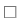false;`

### Carrier and PDSCH Configuration

Set waveform type, PDSCH numerology (subcarrier spacing (SCS) and cyclic prefix (CP) type), and other transmission configuration parameters.

```% SCS carrier parameters simParameters.Carrier = nrCarrierConfig; % Carrier resource grid configuration simParameters.Carrier.NSizeGrid = 52; % Bandwidth in number of resource blocks simParameters.Carrier.SubcarrierSpacing = 15; % 15, 30, 60, 120 (kHz) simParameters.Carrier.CyclicPrefix = 'Normal'; % 'Normal' or 'Extended' (Extended CP is relevant for 60 kHz SCS only) simParameters.Carrier.NCellID = 1; % Cell identity```

Specify a full-band slot-wise PDSCH transmission and reserve the first 2 OFDM symbols.

```simParameters.PDSCH = nrPDSCHConfig; % This PDSCH definition is the basis for all PDSCH transmissions in the simulation % Define PDSCH time allocation in a slot simParameters.PDSCH.MappingType =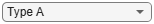"A"; % PDSCH mapping type ('A'(slot-wise),'B'(non slot-wise)) symAlloc = [2 simParameters.Carrier.SymbolsPerSlot-2]; % Starting symbol and number of symbols of each PDSCH allocation simParameters.PDSCH.SymbolAllocation = symAlloc; % Define PDSCH frequency resource allocation per slot to be full grid simParameters.PDSCH.PRBSet = 0:simParameters.Carrier.NSizeGrid-1; % Scrambling identifiers simParameters.PDSCH.NID = simParameters.Carrier.NCellID; simParameters.PDSCH.RNTI = 1;```

Specify the DM-RS configuration, as defined in TS 38.211 Section 7.4.1.1. The DM-RS port set is dynamic and based on CSI feedback (RI).

```simParameters.PDSCH.DMRS.DMRSTypeAPosition =2; % Mapping type A only. First DM-RS symbol position (2,3) simParameters.PDSCH.DMRS.DMRSLength =1; % Number of front-loaded DM-RS symbols (1(single symbol),2(double symbol)) simParameters.PDSCH.DMRS.DMRSAdditionalPosition =2; % Additional DM-RS symbol positions (max range 0...3) simParameters.PDSCH.DMRS.DMRSConfigurationType =2; % DM-RS configuration type (1,2) simParameters.PDSCH.DMRS.NumCDMGroupsWithoutData =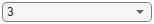3; % CDM groups without data (1,2,3)```

Specify additional simulation parameters for the DL-SCH and PDSCH. Set the frequency granularity of the MIMO precoder, as defined in TS 38.214 Section 5.1.2.3, the modulation and coding scheme table, as defined in TS 38.214 Section 5.1.3.1, and the overhead for transport block size calculation. Set `XOverhead` to empty (`[]`) for automatic selection based on the CSI-RS allocated REs.

```simParameters.PDSCHExtension = struct(); simParameters.PDSCHExtension.PRGBundleSize =4; % 2, 4, or [] to signify "wideband" simParameters.PDSCHExtension.MCSTable ='Table1'; % 'Table1',...,'Table4' simParameters.PDSCHExtension.XOverhead =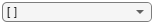[ ]; % 0, 6, 12, 18, or [] for automatic selection.```

Configure the LDPC decoding algorithm and maximum LDPC iterations. The available decoding algorithms are '`Belief propagation`', '`Layered belief propagation`', '`Normalized min-sum`', and '`Offset min-sum`'.

```simParameters.PDSCHExtension.LDPCDecodingAlgorithm =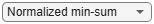"Normalized min-sum"; simParameters.PDSCHExtension.MaximumLDPCIterationCount = 6;```

### Antenna Panel Configuration

Configure the antenna panel geometry by specifying the number of antenna panels (${\mathit{N}}_{\mathit{g}}$) and their dimensions (${\mathit{N}}_{1}$ and ${\mathit{N}}_{2}$). ${\mathit{N}}_{1}$ and ${\mathit{N}}_{2}$ are the number of cross-polarized antenna elements in horizontal and vertical directions for each panel, respectively, and ${\mathit{N}}_{\mathit{g}}$ is the number of column array panels. This diagram shows an antenna panel arrangement and its dimensions.The MIMO precoding codebooks defined in TS 38.214 Section 5.2.2.2 restrict the values of ${\mathit{N}}_{\mathit{g}}$, ${\mathit{N}}_{1}$, and ${\mathit{N}}_{2}$ that 5G NR supports. To configure single-input single-output (SISO) transmissions with single-polarized antenna elements, set the panel dimensions to [1 1]. The receiver panel dimensions are not limited by the codebooks.

```simParameters.TransmitAntennaArray.NumPanels =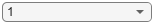1; % Number of panels in horizontal dimension (Ng) simParameters.TransmitAntennaArray.PanelDimensions =[2 2]; % Number of columns and rows in a panel (N1, N2) simParameters.ReceiveAntennaArray.NumPanels =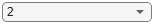1; % Number of panels in horizontal dimension (Ng) simParameters.ReceiveAntennaArray.PanelDimensions = [2 1]; % Number of columns and rows in a panel (N1, N2) ```

Due to polarization, the overall number of antennas is $2×{\mathit{N}}_{1}×{\mathit{N}}_{2}×{\mathit{N}}_{\mathit{g}}$.

```simParameters.NTxAnts = numAntennaElements(simParameters.TransmitAntennaArray); simParameters.NRxAnts = numAntennaElements(simParameters.ReceiveAntennaArray);```

### CSI-RS Configuration

The simulation configures an 8-port CSI-RS, which maps to each antenna element of the transmit panel. The number of CSI-RS ports must match the transmit array panel's dimensions, as defined in TS 38.214 Table 5.2.2.2.1-2 for single-panel and Table 5.2.2.2.2-1 for multi-panel codebooks. These tables show what CSI-RS row numbers are compatible with the selected array panel dimensions.Configure the CSI-RS transmission parameters.

```simParameters.CSIRS = nrCSIRSConfig; simParameters.CSIRS.CSIRSType ='nzp'; % 'nzp','zp' simParameters.CSIRS.RowNumber =6; % 1...18 simParameters.CSIRS.NumRB = simParameters.Carrier.NSizeGrid - simParameters.CSIRS.RBOffset; simParameters.CSIRS.CSIRSPeriod = [10 0]; simParameters.CSIRS.SymbolLocations = 4; simParameters.CSIRS.SubcarrierLocations = [0,3,6,9]; simParameters.CSIRS.Density = 'one'; disp(['Number of CSI-RS ports: ' num2str(simParameters.CSIRS.NumCSIRSPorts) '.'])```
```Number of CSI-RS ports: 8. ```
```csirsCDMLengths = getCSIRSCDMLengths(simParameters.CSIRS); % Check that the number of CSI-RS ports and transmit antenna elements match % and the consistency of multiple CSI-RS resources validateCSIRSConfig(simParameters.Carrier,simParameters.CSIRS,simParameters.NTxAnts);```

### CSI Feedback Configuration

Specify the CSI feedback mode as one of '`RI-PMI-CQI`', '`AI CSI compression`', or '`Perfect CSI`'. In all modes, the UE can perform perfect or practical channel estimation using CSI-RS.

• RI-PMI-CQI: The UE selects and reports the appropriate RI, PMI, and CQI periodically. The gNodeB follows the reported CSI to configure the target code rate, modulation, number of layers, and MIMO precoding matrix in subsequent transmissions after a configurable delay.

• AI CSI compression: The UE compresses channel estimates using an AI neural network autoencoder and reports them to the gNodeB periodically. The gNodeB recovers the channel estimates and selects appropriate target code rate, modulation, number of layers, and MIMO precoding matrix. The gNodeB applies the selected configuration in a subsequent PDSCH transmission after a configurable delay. You can specify the filename of the neural network. This simulation downloads a pretrained neural network that is valid only when the number of transmit antennas is 8 and a carrier grid size of 52 RB and 15 kHz subcarrier spacing. For other number of transmit antennas and bandwidths, you need to train the neural network as described in CSI Feedback with Autoencoders.

• Perfect CSI: The UE reports channel estimates to the gNodeB periodically. This mode is equivalent to the '`AI CSI compression`' mode without compression losses.

`simParameters.CSIReportMode ='RI-PMI-CQI'; % 'RI-PMI-CQI','AI CSI compression','Perfect CSI'`

Specify the CSI report configuration. For more information on the CSI report configuration, see the 5G NR Downlink CSI Reporting example.

```simParameters.CSIReportConfig = struct(); simParameters.CSIReportConfig.Period = [5 0]; % Peridocity and offset of the CSI report in slots if simParameters.CSIReportMode == "RI-PMI-CQI" simParameters.CSIReportConfig.CQITable ="Table1"; % 'Table1','Table2','Table3' simParameters.CSIReportConfig.CQIMode ='Wideband'; % 'Wideband','Subband' simParameters.CSIReportConfig.PMIMode ='Subband'; % 'Wideband','Subband' simParameters.CSIReportConfig.CodebookType ='Type1SinglePanel'; % 'Type1SinglePanel','Type1MultiPanel','Type2' simParameters.CSIReportConfig.SubbandSize =4; % Subband size in RB (4,8,16,32) simParameters.CSIReportConfig.CodebookMode =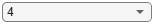1; % 1,2 simParameters.CSIReportConfig.RIRestriction = []; % Empty for no rank restriction simParameters.CSIReportConfig.NumberOfBeams =2; % 2,3,4. Only for Type II codebooks simParameters.CSIReportConfig.PhaseAlphabetSize =8; % 4,8. Only for Type II codebooks simParameters.CSIReportConfig.SubbandAmplitude =true; % true/false. Only for Type II codebooks simParameters.CSIReportConfig.NStartBWP = []; % Empty to signal the entire carrier simParameters.CSIReportConfig.NSizeBWP = []; % Empty to signal the entire carrier % Configure the CSI report with the antenna panel dimensions specified simParameters.CSIReportConfig.PanelDimensions = getCSIReportPanelDimensions(simParameters.TransmitAntennaArray,simParameters.CSIReportConfig.CodebookType); else % AI CSI compression % Specify the file name of the AI neural network simParameters.AINetworkFilename = 'csiTrainedNetwork.mat'; end```

Configure the CSI processing delay in slots. For the UE, this delay is the number of time slots between the reception of the CSI-RS and the availability of the CSI feedback. For the gNodeB, the delay is the number of time slots between the reception of the CSI report and the transmission using the recommended CSI.

```simParameters.UEProcessingDelay = 7; simParameters.BSProcessingDelay = 1;```

### Propagation Channel Configuration

Configure the delay profile, delay spread, and maximum Doppler shift of the propagation channel for the simulation. Both CDL and TDL channel models are supported. The panel dimensions and cross-polarized elements specified in Antenna Panel Configuration define the geometry of the antenna arrays for CDL channels.

```simParameters.DelayProfile = 'CDL-C'; % 'CDL-A',...,'CDL-E','TDL-A',...,'TDL-E' simParameters.DelaySpread = 300e-9; % s simParameters.MaximumDopplerShift = 5; % Hz simParameters.Channel = createChannel(simParameters);```

### Processing Loop

To determine the PDSCH throughput for each SNR point, the simulation performs these steps:

1. Update DL-SCH and PDSCH transmission parameters (target code rate, number of layers, modulation, and MIMO precoding matrix). This step applies only when a new CSI report is available.

2. Map CSI-RS signals to the resource grid.

3. Perform channel coding (DL-SCH) and PDSCH encoding. Map PDSCH and PDSCH DM-RS to the resource grid.

4. OFDM modulate the generated grid.

5. Pass the signal through a fading channel with AWGN.

6. Perform synchronization and OFDM demodulation.

7. Perform PDSCH DM-RS based channel estimation.

8. Perform equalization.

9. Decode the PDSCH and DL-SCH, and measure the PDSCH throughput.

10. Perform CSI-RS based channel estimation.

11. Create CSI report.

12. Feed the CSI report back to the transmitter with the appropriate delay.

To reduce the total simulation time, you can execute the SNR loop in parallel by using the Parallel Computing Toolbox™. Comment out the `for` statement and uncomment the `parfor` statement. If the Parallel Computing Toolbox is not installed, `parfor` defaults to normal `for` statement. Because parfor-loop iterations are executed in parallel in a nondeterministic order, the simulation information displayed for each SNR point can be intertwined. To switch off simulation information display, set the `displaySimulationInformation` variable above to false.

```% Array to store the maximum throughput for all SNR points maxThroughput = zeros(length(simParameters.SNRIn),1); % Array to store the simulation throughput for all SNR points simThroughput = zeros(length(simParameters.SNRIn),1); % Cell array to store CSI reports per SNR point CSIReport = {}; for snrIdx = 1:numel(simParameters.SNRIn) % parfor snrIdx = 1:numel(simParameters.SNRIn) % To reduce the total simulation time, you can execute this loop in % parallel by using the Parallel Computing Toolbox. Comment out the 'for' % statement and uncomment the 'parfor' statement. % Reset the random number generator for repeatability rng(0,"twister"); % Display simulation information at this SNR point displaySNRPointProgress(simParameters,snrIdx); % Take full copies of the simulation-level parameter structures so that % they are not PCT broadcast variables when using parfor simParamLocal = simParameters; % Set up the transmitter, propagation channel, receiver, and CSI % feedback configuration parameters. [carrier,encodeDLSCH,pdsch,pdschextra,csirs,wtx] = setupTransmitter(simParamLocal); [channel,maxChDelay,N0] = setupChannel(simParamLocal,snrIdx); [decodeDLSCH,pathFilters,timingOffset] = setupReceiver(simParamLocal); csiFeedbackOpts = getCSIFeedbackOptions(simParamLocal,snrIdx); % Obtain an initial CSI report based on perfect channel estimates that % the Tx can use to adapt the transmission parameters. csiReports = initialCSIReport(simParamLocal,snrIdx,carrier,csirs,channel,csiFeedbackOpts); csiAvailableSlots = 0; % Total number of slots in the simulation period NSlots = simParamLocal.NFrames * carrier.SlotsPerFrame; % Loop over the entire waveform length for nslot = 0:NSlots-1 % Update the carrier slot numbers for new slot carrier.NSlot = nslot; % Use new CSI report to configure the number of layers and % modulation of the PDSCH and target code rate of the DL-SCH if % there is a new report available. [isNewReport,repIdx] = ismember(nslot,csiAvailableSlots); if isNewReport [pdsch.Modulation,pdschextra.TargetCodeRate,wtx] = hCSIDecode(carrier,pdsch,pdschextra,csiReports(repIdx),csiFeedbackOpts); pdsch.NumLayers = size(wtx,1); encodeDLSCH.TargetCodeRate = pdschextra.TargetCodeRate; end % Create an OFDM resource grid for a slot dlGrid = nrResourceGrid(carrier,csirs.NumCSIRSPorts); % CSI-RS mapping to the slot resource grid [csirsInd,csirsInfo] = nrCSIRSIndices(carrier,csirs); csirsSym = nrCSIRS(carrier,csirs); dlGrid(csirsInd) = csirsSym; csirsTransmission = ~isempty(csirsInd); % PDSCH reserved REs for CSI-RS pdsch.ReservedRE = csirsInd-1; % 0-based indices % PDSCH generation % Calculate the transport block sizes for the transmission in the slot [pdschIndices,pdschIndicesInfo] = nrPDSCHIndices(carrier,pdsch); trBlkSizes = nrTBS(pdsch.Modulation,pdsch.NumLayers,numel(pdsch.PRBSet),pdschIndicesInfo.NREPerPRB,pdschextra.TargetCodeRate,pdschextra.XOverhead); % Transport block generation for cwIdx = 1:pdsch.NumCodewords % New data for current codeword then create a new DL-SCH transport block trBlk = randi([0 1],trBlkSizes(cwIdx),1); setTransportBlock(encodeDLSCH,trBlk,cwIdx-1); resetSoftBuffer(decodeDLSCH,cwIdx-1); end % Encode the DL-SCH transport blocks RV = zeros(1,pdsch.NumCodewords); codedTrBlocks = encodeDLSCH(pdsch.Modulation,pdsch.NumLayers, ... pdschIndicesInfo.G,RV); % PDSCH modulation and precoding pdschSymbols = nrPDSCH(carrier,pdsch,codedTrBlocks); [pdschAntSymbols,pdschAntIndices] = nrPDSCHPrecode(carrier,pdschSymbols,pdschIndices,wtx); dlGrid(pdschAntIndices) = pdschAntSymbols; % PDSCH DM-RS precoding and mapping dmrsSymbols = nrPDSCHDMRS(carrier,pdsch); dmrsIndices = nrPDSCHDMRSIndices(carrier,pdsch); [dmrsAntSymbols,dmrsAntIndices] = nrPDSCHPrecode(carrier,dmrsSymbols,dmrsIndices,wtx); dlGrid(dmrsAntIndices) = dmrsAntSymbols; % OFDM modulation txWaveform = nrOFDMModulate(carrier,dlGrid); % Pass data through channel model. Append zeros at the end of the % transmitted waveform to flush channel content. These zeros take % into account any delay introduced in the channel. txWaveform = [txWaveform; zeros(maxChDelay,size(txWaveform,2))]; %#ok<AGROW> [rxWaveform,pathGains,sampleTimes] = channel(txWaveform); % Add AWGN to the received time-domain waveform noise = N0*complex(randn(size(rxWaveform)),randn(size(rxWaveform))); rxWaveform = rxWaveform + noise; if (simParamLocal.PerfectChannelEstimator) % Perfect synchronization. Use information provided by the % channel to find the strongest multipath component pathFilters = getPathFilters(channel); [timingOffset,mag] = nrPerfectTimingEstimate(pathGains,pathFilters); else % Practical synchronization. Correlate the received waveform % with the PDSCH DM-RS to obtain the timing offset and % correlation magnitude. The receiver updates the timing offset % only when the correlation magnitude is high. [t,mag] = nrTimingEstimate(carrier,rxWaveform,dmrsIndices,dmrsSymbols); timingOffset = hSkipWeakTimingOffset(timingOffset,t,mag); % Display a warning if the estimated timing offset exceeds the % maximum channel delay if timingOffset > maxChDelay warning(['Estimated timing offset (%d) is greater than the maximum channel delay (%d).' ... ' This will result in a decoding failure. This may be caused by low SNR,' ... ' or not enough DM-RS symbols to synchronize successfully.'],timingOffset,maxChDelay); end end rxWaveform = rxWaveform(1+timingOffset:end,:); % Perform OFDM demodulation on the received data to recreate the % resource grid, including padding in the event that practical % synchronization results in an incomplete slot being demodulated rxGrid = nrOFDMDemodulate(carrier,rxWaveform); [K,L,R] = size(rxGrid); if (L < carrier.SymbolsPerSlot) rxGrid = cat(2,rxGrid,zeros(K,carrier.SymbolsPerSlot-L,R)); end if (simParamLocal.PerfectChannelEstimator) % Perfect channel estimation, using the value of the path gains % provided by the channel. This channel estimate does not % include the effect of transmitter precoding Hest = nrPerfectChannelEstimate(carrier,pathGains,pathFilters,timingOffset,sampleTimes); % Get perfect noise estimate (from the noise realization) noiseGrid = nrOFDMDemodulate(carrier,noise(1+timingOffset:end ,:)); noiseEst = var(noiseGrid(:)); % Get PDSCH resource elements from the received grid and % channel estimate [pdschRx,pdschHest,~,pdschHestIndices] = nrExtractResources(pdschIndices,rxGrid,Hest); % Apply precoding to channel estimate pdschHest = nrPDSCHPrecode(carrier,pdschHest,pdschHestIndices,permute(wtx,[2 1 3])); else % Practical channel estimation between the received grid and % each transmission layer, using the PDSCH DM-RS for each % layer. This channel estimate includes the effect of % transmitter precoding [Hest,noiseEst] = hSubbandChannelEstimate(carrier,rxGrid,dmrsIndices,dmrsSymbols,pdschextra.PRGBundleSize,'CDMLengths',pdsch.DMRS.CDMLengths); % Average noise estimate across PRGs and layers noiseEst = mean(noiseEst,'all'); % Get PDSCH resource elements from the received grid and % channel estimate [pdschRx,pdschHest] = nrExtractResources(pdschIndices,rxGrid,Hest); end % Equalization [pdschEq,eqCSIScaling] = nrEqualizeMMSE(pdschRx,pdschHest,noiseEst); % Decode PDSCH physical channel [dlschLLRs,rxSymbols] = nrPDSCHDecode(carrier,pdsch,pdschEq,noiseEst); % Display EVM per layer, per slot and per RB if (simParamLocal.DisplayDiagnostics) plotLayerEVM(NSlots,nslot,pdsch,size(dlGrid),pdschIndices,pdschSymbols,pdschEq); end % Scale LLRs eqCSIScaling = nrLayerDemap(eqCSIScaling); % CSI scaling layer demapping for cwIdx = 1:pdsch.NumCodewords Qm = length(dlschLLRs{cwIdx})/length(rxSymbols{cwIdx}); % bits per symbol eqCSIScaling{cwIdx} = repmat(eqCSIScaling{cwIdx}.',Qm,1); % expand by each bit per symbol dlschLLRs{cwIdx} = dlschLLRs{cwIdx} .* eqCSIScaling{cwIdx}(:); % scale LLRs end % Decode the DL-SCH transport channel decodeDLSCH.TransportBlockLength = trBlkSizes; decodeDLSCH.TargetCodeRate = pdschextra.TargetCodeRate; [decbits,blkerr] = decodeDLSCH(dlschLLRs,pdsch.Modulation,pdsch.NumLayers,RV); % Store values to calculate throughput simThroughput(snrIdx) = simThroughput(snrIdx) + sum(~blkerr .* trBlkSizes); maxThroughput(snrIdx) = maxThroughput(snrIdx) + sum(trBlkSizes); % CSI measurements and encoding if csirsTransmission if (~simParamLocal.PerfectChannelEstimator) % Consider only the NZP-CSI-RS symbols and indices for CSI-RS based % channel estimation nzpind = (csirsSym ~= 0); % Calculate practical channel estimate based on CSI-RS. Use % a time-averaging window that covers all of the % transmitted CSI-RS symbols. [Hest,noiseEst] = nrChannelEstimate(carrier,rxGrid, ... csirsInd(nzpind),csirsSym(nzpind),'CDMLengths',csirsCDMLengths); end % Generate CSI report. Store the report for use at the % transmitter. The CSI feedback is subject to a delay that % depends on the CSI report periodicity and the UE processing % delay. The slot in which the CSI is available to use at the % transmitter depends on the BS processing delay as well. rxCSIReport = hCSIEncode(carrier,csirs,Hest,noiseEst,csiFeedbackOpts); csiFeedbackSlot = nextCSISlot(csiFeedbackOpts.CSIReportPeriod,1+nslot+simParamLocal.UEProcessingDelay); csiAvailableSlots(end+1) = 1+csiFeedbackSlot+simParamLocal.BSProcessingDelay; %#ok<SAGROW> csiReports(end+1) = rxCSIReport; %#ok<SAGROW> end % Print slot-wise information if (simParamLocal.DisplaySimulationInformation) printSlotInfo(NSlots,carrier,pdsch,pdschextra,blkerr,trBlkSizes./pdschIndicesInfo.G,csirsTransmission,csiReports,repIdx) end end % Store CSI report for each SNR point CSIReport{snrIdx} = csiReports; %#ok<SAGROW> % Display the results dynamically in the command window if (simParamLocal.DisplaySimulationInformation) fprintf('\n'); end fprintf('\nThroughput(Mbps) for %d frame(s) = %.4f\n',simParamLocal.NFrames,1e-6*simThroughput(snrIdx)/(simParamLocal.NFrames*10e-3)); end```
```Simulating transmission scheme 1 (8x4) and SCS=15kHz with CDL-C channel at -10dB SNR for 2 10ms frame(s) Using RI, PMI, and CQI as CSI feedback. ```
```( 5.00%) NSlot= 0: Transmission succeeded (Layers=1, Mod=16QAM, TCR=0.479, CR=0.456). Using initial CSI. CSI-RS transmission. (10.00%) NSlot= 1: Transmission succeeded (Layers=1, Mod=16QAM, TCR=0.479, CR=0.422). (15.00%) NSlot= 2: Transmission succeeded (Layers=1, Mod=16QAM, TCR=0.479, CR=0.422). (20.00%) NSlot= 3: Transmission succeeded (Layers=1, Mod=16QAM, TCR=0.479, CR=0.422). (25.00%) NSlot= 4: Transmission succeeded (Layers=1, Mod=16QAM, TCR=0.479, CR=0.422). (30.00%) NSlot= 5: Transmission succeeded (Layers=1, Mod=16QAM, TCR=0.479, CR=0.422). (35.00%) NSlot= 6: Transmission succeeded (Layers=1, Mod=16QAM, TCR=0.479, CR=0.422). (40.00%) NSlot= 7: Transmission succeeded (Layers=1, Mod=16QAM, TCR=0.479, CR=0.422). (45.00%) NSlot= 8: Transmission succeeded (Layers=1, Mod=16QAM, TCR=0.479, CR=0.422). (50.00%) NSlot= 9: Transmission succeeded (Layers=1, Mod=16QAM, TCR=0.479, CR=0.422). (55.00%) NSlot=10: Transmission succeeded (Layers=1, Mod=16QAM, TCR=0.479, CR=0.456). CSI-RS transmission. (60.00%) NSlot=11: Transmission succeeded (Layers=1, Mod=16QAM, TCR=0.479, CR=0.422). (65.00%) NSlot=12: Transmission succeeded (Layers=1, Mod=16QAM, TCR=0.479, CR=0.422). Using CSI from NSlot= 0. (70.00%) NSlot=13: Transmission succeeded (Layers=1, Mod=16QAM, TCR=0.479, CR=0.422). (75.00%) NSlot=14: Transmission succeeded (Layers=1, Mod=16QAM, TCR=0.479, CR=0.422). (80.00%) NSlot=15: Transmission succeeded (Layers=1, Mod=16QAM, TCR=0.479, CR=0.422). (85.00%) NSlot=16: Transmission succeeded (Layers=1, Mod=16QAM, TCR=0.479, CR=0.422). (90.00%) NSlot=17: Transmission succeeded (Layers=1, Mod=16QAM, TCR=0.479, CR=0.422). (95.00%) NSlot=18: Transmission succeeded (Layers=1, Mod=16QAM, TCR=0.479, CR=0.422). (100.00%) NSlot=19: Transmission succeeded (Layers=1, Mod=16QAM, TCR=0.479, CR=0.422). ```
```Throughput(Mbps) for 2 frame(s) = 9.4800 ```
```Simulating transmission scheme 1 (8x4) and SCS=15kHz with CDL-C channel at 10dB SNR for 2 10ms frame(s) Using RI, PMI, and CQI as CSI feedback. ```
```( 5.00%) NSlot= 0: Transmission succeeded (Layers=3, Mod=64QAM, TCR=0.650, CR=0.624). Using initial CSI. CSI-RS transmission. (10.00%) NSlot= 1: Transmission succeeded (Layers=3, Mod=64QAM, TCR=0.650, CR=0.578). (15.00%) NSlot= 2: Transmission succeeded (Layers=3, Mod=64QAM, TCR=0.650, CR=0.578). (20.00%) NSlot= 3: Transmission succeeded (Layers=3, Mod=64QAM, TCR=0.650, CR=0.578). (25.00%) NSlot= 4: Transmission succeeded (Layers=3, Mod=64QAM, TCR=0.650, CR=0.578). (30.00%) NSlot= 5: Transmission succeeded (Layers=3, Mod=64QAM, TCR=0.650, CR=0.578). (35.00%) NSlot= 6: Transmission succeeded (Layers=3, Mod=64QAM, TCR=0.650, CR=0.578). (40.00%) NSlot= 7: Transmission succeeded (Layers=3, Mod=64QAM, TCR=0.650, CR=0.578). (45.00%) NSlot= 8: Transmission succeeded (Layers=3, Mod=64QAM, TCR=0.650, CR=0.578). (50.00%) NSlot= 9: Transmission succeeded (Layers=3, Mod=64QAM, TCR=0.650, CR=0.578). (55.00%) NSlot=10: Transmission succeeded (Layers=3, Mod=64QAM, TCR=0.650, CR=0.624). CSI-RS transmission. (60.00%) NSlot=11: Transmission succeeded (Layers=3, Mod=64QAM, TCR=0.650, CR=0.578). (65.00%) NSlot=12: Transmission succeeded (Layers=3, Mod=64QAM, TCR=0.650, CR=0.578). Using CSI from NSlot= 0. (70.00%) NSlot=13: Transmission succeeded (Layers=3, Mod=64QAM, TCR=0.650, CR=0.578). (75.00%) NSlot=14: Transmission succeeded (Layers=3, Mod=64QAM, TCR=0.650, CR=0.578). (80.00%) NSlot=15: Transmission succeeded (Layers=3, Mod=64QAM, TCR=0.650, CR=0.578). (85.00%) NSlot=16: Transmission succeeded (Layers=3, Mod=64QAM, TCR=0.650, CR=0.578). (90.00%) NSlot=17: Transmission succeeded (Layers=3, Mod=64QAM, TCR=0.650, CR=0.578). (95.00%) NSlot=18: Transmission succeeded (Layers=3, Mod=64QAM, TCR=0.650, CR=0.578). (100.00%) NSlot=19: Transmission succeeded (Layers=3, Mod=64QAM, TCR=0.650, CR=0.578). ```
```Throughput(Mbps) for 2 frame(s) = 58.3840 ```

### Results

Display the measured throughput as a function of the SNR.

```figure; plot(simParameters.SNRIn,1e-6*simThroughput/(simParameters.NFrames*10e-3),'o-.') xlabel('SNR (dB)'); ylabel('Throughput (Mbps)'); grid on; title(sprintf('%s (%dx%d) / NRB=%d / SCS=%dkHz / CSI: %s', ... simParameters.DelayProfile,simParameters.NTxAnts,simParameters.NRxAnts, ... simParameters.Carrier.NSizeGrid,simParameters.Carrier.SubcarrierSpacing,... char(simParameters.CSIReportMode)));```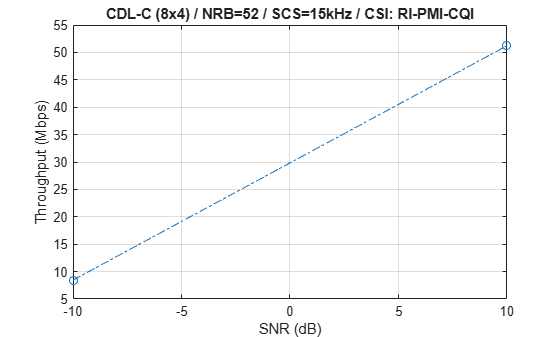```if simParameters.CSIReportMode == "RI-PMI-CQI" perc = 90; plotCQI(simParameters,CSIReport,perc) end``````% Bundle key parameters and results into a combined structure for recording simResults.simParameters = simParameters; simResults.simThroughput = simThroughput; simResults.maxThroughput = maxThroughput; simResults.CSIReport = CSIReport;```

### Local Functions

```function [carrier,eDLSCH,pdsch,pdschextra,csirs,wtx] = setupTransmitter(simParameters) % Extract channel and signal-level parameters, create DL-SCH encoder, and % initialize MIMO precoding matrix. carrier = simParameters.Carrier; pdsch = simParameters.PDSCH; pdschextra = simParameters.PDSCHExtension; csirs = simParameters.CSIRS; % Select XOverhead for TBS calculation if required if isempty(pdschextra.XOverhead) pdschextra.XOverhead = getXOverhead(carrier,csirs); end % Create DL-SCH encoder system object to perform transport channel % encoding eDLSCH = nrDLSCH; % Initialize MIMO precoding matrix wtx = 1; end function [decodeDLSCH,pathFilters,timingOffset] = setupReceiver(simParameters) % Create and configure DL-SCH decoder and initialize receiver parameters % Create DL-SCH decoder system object to perform transport channel % decoding decodeDLSCH = nrDLSCHDecoder; decodeDLSCH.LDPCDecodingAlgorithm = simParameters.PDSCHExtension.LDPCDecodingAlgorithm; decodeDLSCH.MaximumLDPCIterationCount = simParameters.PDSCHExtension.MaximumLDPCIterationCount; % Initialize channel path filters and timing offset. Timing offset is % updated in every slot for perfect synchronization and when the % correlation is strong for practical synchronization pathFilters = []; timingOffset = 0; end function channel = createChannel(simParameters) % Create and configure the propagation channel. If the number of antennas % is 1, configure only 1 polarization, otherwise configure 2 polarizations. % Number of antenna elements and polarizations nTxAnts = simParameters.NTxAnts; numTxPol = 1 + (nTxAnts>1); nRxAnts = simParameters.NRxAnts; numRxPol = 1 + (nRxAnts>1); if contains(simParameters.DelayProfile,'CDL') % Create CDL channel channel = nrCDLChannel; % Tx antenna array configuration in CDL channel. The number of antenna % elements depends on the panel dimensions. The size of the antenna % array is [M,N,P,Mg,Ng]. M and N are the number of rows and columns in % the antenna array. P is the number of polarizations (1 or 2). Mg and % Ng are the number of row and column array panels respectively. Note % that N1 and N2 in the panel dimensions follow a different convention % and denote the number of columns and rows, respectively. txArray = simParameters.TransmitAntennaArray; M = txArray.PanelDimensions(2); N = txArray.PanelDimensions(1); Ng = txArray.NumPanels; channel.TransmitAntennaArray.Size = [M N numTxPol 1 Ng]; channel.TransmitAntennaArray.ElementSpacing = [0.5 0.5 1 1]; % Element spacing in wavelengths channel.TransmitAntennaArray.PolarizationAngles = [-45 45]; % Polarization angles in degrees % Rx antenna array configuration in CDL channel rxArray = simParameters.ReceiveAntennaArray; M = rxArray.PanelDimensions(2); N = rxArray.PanelDimensions(1); Ng = rxArray.NumPanels; channel.ReceiveAntennaArray.Size = [M N numRxPol 1 Ng]; channel.ReceiveAntennaArray.ElementSpacing = [0.5 0.5 1 1]; % Element spacing in wavelengths channel.ReceiveAntennaArray.PolarizationAngles = [0 90]; % Polarization angles in degrees elseif contains(simParameters.DelayProfile,'TDL') channel = nrTDLChannel; channel.NumTransmitAntennas = nTxAnts; channel.NumReceiveAntennas = nRxAnts; else error('Channel not supported.') end % Configure common channel parameters: delay profile, delay spread, and % maximum Doppler shift channel.DelayProfile = simParameters.DelayProfile; channel.DelaySpread = simParameters.DelaySpread; channel.MaximumDopplerShift = simParameters.MaximumDopplerShift; % Get information about the baseband waveform after OFDM modulation step waveInfo = nrOFDMInfo(simParameters.Carrier); % Update channel sample rate based on carrier information channel.SampleRate = waveInfo.SampleRate; end function [channel,maxChannelDelay,N0] = setupChannel(simParameters,snrIdx) % Reset the propagation channel. Obtain the maximum channel % delay and calculate the AWGN standard deviation. % Extract channel channel = simParameters.Channel; channel.reset(); % Get the channel information chInfo = info(channel); maxChannelDelay = chInfo.MaximumChannelDelay; % Calculate noise standard deviation. Normalize noise power by the IFFT % size used in OFDM modulation, as the OFDM modulator applies this % normalization to the transmitted waveform. SNRdB = simParameters.SNRIn(snrIdx); SNR = 10^(SNRdB/10); waveInfo = nrOFDMInfo(simParameters.Carrier); N0 = 1/sqrt(2.0*double(waveInfo.Nfft)*SNR); % Also normalize by the number of receive antennas if the channel % applies this normalization to the output if channel.NormalizeChannelOutputs N0 = N0/sqrt(chInfo.NumOutputSignals); end end function csiReport = initialCSIReport(simParameters,snrIdx,carrier,csirs,channel,csiFeedbackOpts) % Get an initial CSI report using a perfect channel estimate [Hest,nVar] = getInitialChannelEstimate(carrier,channel,simParameters.SNRIn(snrIdx)); csiFeedbackOpts.PerfectChannelEstimator = true; csirs.CSIRSPeriod = 'on'; csiReport = hCSIEncode(carrier,csirs,Hest,nVar,csiFeedbackOpts); end function [estChannelGrid,nVar] = getInitialChannelEstimate(carrier,propchannel,SNRdB) % Obtain channel estimate before first transmission. This can be used to % obtain initial transmission parameters ofdmInfo = nrOFDMInfo(carrier); chInfo = info(propchannel); % Clone channel and get path gains and sample times for perfect timing % and channel estimation channel = clone(propchannel); release(channel); channel.ChannelFiltering = false; channel.NumTimeSamples = (ofdmInfo.SampleRate/1000/carrier.SlotsPerSubframe)+chInfo.MaximumChannelDelay; [pathGains,sampleTimes] = channel(); % Perfect timing synch pathFilters = getPathFilters(channel); offset = nrPerfectTimingEstimate(pathGains,pathFilters); % Perfect channel estimate estChannelGrid = nrPerfectChannelEstimate(carrier,pathGains,pathFilters,offset,sampleTimes); % Noise variance (does not account for channel effects at this point.) nVar = 10^(-SNRdB/10)/size(estChannelGrid,3); end function XOverhead = getXOverhead(carrier,csirs) % Calculate XOverhead for transport block size determination based on % CSI-RS resource grid occupancy [~,csirsInfo] = nrCSIRSIndices(carrier,csirs); csirsRE = length(csirsInfo.KBarLBar{1})*length(csirsInfo.KPrime{1})*length(csirsInfo.LPrime{1}); [~,XOverhead] = quantiz(csirsRE,[0 6 12],[0 6 12 18]); end function cdmLengths = getCSIRSCDMLengths(csirs) % CDMLENGTHS = getCSIRSCDMLengths(CSIRS) returns the CDM lengths given % the CSI-RS configuration object CSIRS. CDMType = csirs.CDMType; if ~iscell(csirs.CDMType) CDMType = {csirs.CDMType}; end CDMTypeOpts = {'noCDM','fd-CDM2','CDM4','CDM8'}; CDMLengthOpts = {[1 1],[2 1],[2 2],[2 4]}; cdmLengths = CDMLengthOpts{strcmpi(CDMTypeOpts,CDMType{1})}; end function csiFeedbackOpts = getCSIFeedbackOptions(simParameters,snrIdx) % Create a CSI feedback algorithmic options structure csiFeedbackOpts = struct(); csiFeedbackOpts.CSIReportMode = simParameters.CSIReportMode; csiFeedbackOpts.CSIReportPeriod = simParameters.CSIReportConfig.Period; csiFeedbackOpts.CSIReportConfig = simParameters.CSIReportConfig; csiFeedbackOpts.PerfectChannelEstimator = simParameters.PerfectChannelEstimator; if simParameters.CSIReportMode == "AI CSI compression" % Copy additional link adaptation configuration for AI CSI compression mode csiFeedbackOpts.AINetworkFilename = simParameters.AINetworkFilename; % Download and extract a pretrained CSI network for AI CSI compression mode displayProgress = (snrIdx==1); helperCSINetDownloadData(displayProgress); end end function panelDimensions = getCSIReportPanelDimensions(antennaArray,codebookType) % Configure the antenna array dimensions according to TS 38.214 Section % 5.2.2.2 as a vector [N1,N2] for single-panel arrays and a vector % [Ng,N1,N2] for multi-panel arrays. panelDimensions = antennaArray.PanelDimensions; % Add number of panels if codebook type is multi-panel if strcmpi(codebookType,'Type1MultiPanel') panelDimensions = [antennaArray.NumPanels panelDimensions]; end end function csislot = nextCSISlot(period,nslot) % Return the slot number of the first slot where CSI feedback can be % reported according to the CSI report periodicity p = period(1); % Slot periodicity o = period(2); % Slot offset csislot = p*ceil((nslot-o)/p)+o; end function [numElemenets,numPol] = numAntennaElements(antArray) % Calculate number of antenna elements in an antenna array numPol = 1 + any(antArray.PanelDimensions ~= 1); numElemenets = numPol*antArray.NumPanels*prod(antArray.PanelDimensions); end function validateCSIRSConfig(carrier,csirs,nTxAnts) % validateCSIRSConfig(CARRIER,CSIRS,NTXANTS) validates the CSI-RS % configuration, given the carrier specific configuration object CARRIER, % CSI-RS configuration object CSIRS, and the number of transmit antennas % NTXANTS. % Validate the number of CSI-RS ports if ~isscalar(unique(csirs.NumCSIRSPorts)) error('nr5g:InvalidCSIRSPorts',... 'All the CSI-RS resources must be configured to have the same number of CSI-RS ports.'); end % Validate the CSI-RS and TX antenna array configuration if any(csirs.Ports_Options(csirs.RowNumber) ~= nTxAnts) rn = num2str(find(csirs.Ports_Options == nTxAnts),'%3d,'); str = 'The number of CSI-RS ports must be equal to the number of Tx antenna elements. '; str = [str sprintf('For the Tx antenna array size configured, valid CSI-RS row numbers are (%s).',rn(1:end-1))]; error(str) end % Validate the CDM lengths if ~iscell(csirs.CDMType) cdmType = {csirs.CDMType}; else cdmType = csirs.CDMType; end if (~all(strcmpi(cdmType,cdmType{1}))) error('nr5g:InvalidCSIRSCDMTypes',... 'All the CSI-RS resources must be configured to have the same CDM lengths.'); end if nTxAnts ~= csirs.NumCSIRSPorts(1) error('nr5g:InvalidNumTxAnts',['Number of transmit antennas (' num2str(nTxAnts)... ') must be equal to the number of CSI-RS ports (' num2str(csirs.NumCSIRSPorts(1)) ').']); end % Check for the overlap between the CSI-RS indices csirsInd = nrCSIRSIndices(carrier,csirs,"OutputResourceFormat",'cell'); numRes = numel(csirsInd); csirsIndAll = cell(1,numRes); ratioVal = csirs.NumCSIRSPorts(1)/prod(getCSIRSCDMLengths(csirs)); for resIdx = 1:numRes if ~isempty(csirsInd{resIdx}) grid = nrResourceGrid(carrier,csirs.NumCSIRSPorts(1)); [~,tempInd] = nrExtractResources(csirsInd{resIdx},grid); if numel(tempInd)/numel(csirsInd{resIdx}) ~= ratioVal error('nr5g:OverlappedCSIRSREsSingleResource',['CSI-RS indices of resource '... num2str(resIdx) ' must be unique. Try changing the symbol or subcarrier locations.']); end csirsIndAll{resIdx} = tempInd(:); for idx = 1:resIdx-1 overlappedInd = ismember(csirsIndAll{idx},csirsIndAll{resIdx}); if any(overlappedInd) error('nr5g:OverlappedCSIRSREsMultipleResources',['The resource elements of the '... 'configured CSI-RS resources must not overlap. Try changing the symbol or '... 'subcarrier locations of CSI-RS resource ' num2str(idx) ' and resource ' num2str(resIdx) '.']); end end end end end function displaySNRPointProgress(simParameters,snrIdx) % Print SNR point progress str = ['\nSimulating transmission scheme 1 (%dx%d) and '... 'SCS=%dkHz with %s channel at %gdB SNR for %d 10ms frame(s)\n' ... 'Using %s as CSI feedback.\n']; switch simParameters.CSIReportMode case 'RI-PMI-CQI' modeText = 'RI, PMI, and CQI'; case 'AI CSI compression' modeText = 'compressed channel estimates'; otherwise modeText = 'channel estimates'; end SNRdB = simParameters.SNRIn(snrIdx); fprintf(str,simParameters.NTxAnts,simParameters.NRxAnts,simParameters.Carrier.SubcarrierSpacing, ... simParameters.DelayProfile,SNRdB,simParameters.NFrames,modeText); end function printSlotInfo(NSlots,carrier,pdsch,pdschextra,blkerr,ECR,csirsTransmission,csiReports,reportIndex) % Print information about the current slot transmission ncw = pdsch.NumCodewords; cwLayers = floor((pdsch.NumLayers + (0:ncw-1)) / ncw); infoStr = []; for cwIdx = 1:ncw if blkerr infoStrCW = "Transmission failed"; else infoStrCW = "Transmission succeeded"; end infoStrCW = sprintf("%22s (Layers=%d, Mod=%5s, TCR=%.3f, CR=%.3f).",infoStrCW,cwLayers(cwIdx),pdsch.Modulation{cwIdx},pdschextra.TargetCodeRate(cwIdx),ECR(cwIdx)); if (ncw>1) infoStr = sprintf('%s\n%s%s',infoStr,sprintf('CW%d: %s',cwIdx-1),infoStrCW); else infoStr = infoStrCW; end end csirsInfoStr = []; if csirsTransmission csirsInfoStr = "CSI-RS transmission. "; end csifbInfoStr = []; if carrier.NSlot == 0 csifbInfoStr = 'Using initial CSI.'; elseif reportIndex > 0 csifbInfoStr = sprintf("Using CSI from NSlot=%2d.",csiReports(reportIndex).NSlot); end nslot = carrier.NSlot; fprintf('\n(%5.2f%%) NSlot=%2d: %s',100*(nslot+1)/NSlots,nslot,join([infoStr,csifbInfoStr,csirsInfoStr])); end function plotLayerEVM(NSlots,nslot,pdsch,siz,pdschIndices,pdschSymbols,pdschEqSymbols) % Plot EVM information persistent slotEVM; persistent rbEVM persistent evmPerSlot; persistent numLayers; maxNumLayers = 8; if (nslot==0) slotEVM = comm.EVM; rbEVM = comm.EVM; evmPerSlot = NaN(NSlots,maxNumLayers); numLayers = pdsch.NumLayers; figure; else % Keep the maximum number of layers in the legend numLayers = max(numLayers,pdsch.NumLayers); end [Ns,P] = size(pdschEqSymbols); pdschEqSym = zeros(Ns,maxNumLayers); pdschSym = zeros(Ns,maxNumLayers); pdschEqSym(:,1:P) = pdschEqSymbols; pdschSym(:,1:P) = pdschSymbols; evmPerSlot(nslot+1,:) = slotEVM(pdschSym,pdschEqSym); subplot(2,1,1); plot(0:(NSlots-1),evmPerSlot,'o-'); xlabel('Slot number'); ylabel('EVM (%)'); legend("layer " + (1:numLayers),'Location','EastOutside'); title('EVM per layer per slot'); subplot(2,1,2); [k,~,p] = ind2sub(siz,pdschIndices); rbsubs = floor((k-1) / 12); NRB = siz(1) / 12; evmPerRB = NaN(NRB,maxNumLayers); for nu = 1:pdsch.NumLayers for rb = unique(rbsubs).' this = (rbsubs==rb & p==nu); evmPerRB(rb+1,nu) = rbEVM(pdschSym(this),pdschEqSym(this)); end end plot(0:(NRB-1),evmPerRB,'x-'); xlabel('Resource block'); ylabel('EVM (%)'); legend("layer " + (1:numLayers),'Location','EastOutside'); title(['EVM per layer per resource block, slot #' num2str(nslot)]); drawnow; end function plotCQI(simParameters,CSIReport,perc) % Plot CQI median and percentiles % Calculate median and percentiles med = cellfun(@(x) median([x.CQI]), CSIReport); p1 = cellfun(@(x) prctile([x.CQI],50-perc/2), CSIReport); p2 = cellfun(@(x) prctile([x.CQI],50+perc/2), CSIReport); % Calculate the percentage of CQI not in the set {median CQI -1, median CQI, median CQI +1} cqiPerc = cellfun(@(x,y) sum(abs([x.CQI]-y)>1)/length(x),CSIReport,num2cell(med)); figure; subplot(211) errorbar(simParameters.SNRIn,med,p2-p1,'o-.') ylabel('CQI value') title(sprintf('Median CQI and (%g,%g) Percentiles',50-perc/2,50+perc/2)); grid subplot(212) plot(simParameters.SNRIn,cqiPerc,'o-.') xlabel('SNR (dB)') ylabel('%') title('CQI not in set \{median CQI -1, median CQI, median CQI +1\}'); grid end```